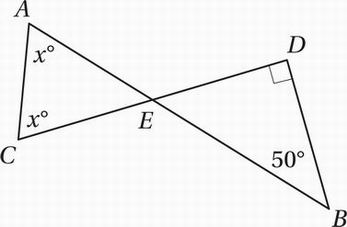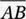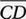# SAT Math Multiple Choice Question 698: Answer and Explanation

### Test Information

Question: 698

8.In the figure above, line segmentsandintersect at point E. What is the value of x?

• A. 60°
• B. 65°
• C. 70°
• D. 75°

Explanation:

C

Special Topics (polygons) EASY

The sum of the measures if the interior angles of a triangle is 180°, therefore mBED + 90° + 50° = 180°, and so mBED = 40°. Since ∠AEC is vertical to ∠BED, it must also have a measure of 40°, and so

40 + x + x = 180

Simplify:

40 + 2x = 180

Subtract 40:

2x = 140

Divide by 2:

x = 70